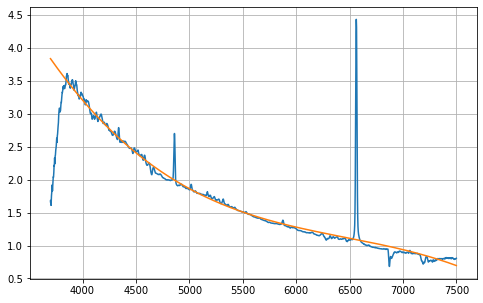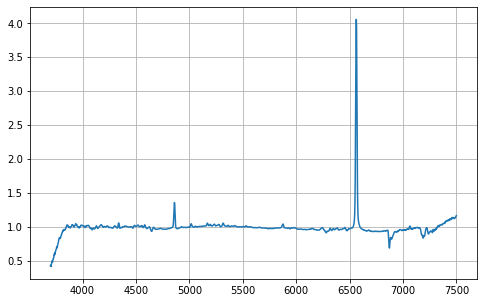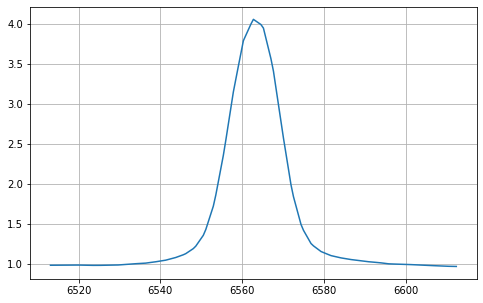#### 4.3 - Spectrum normalization by its continuum¶

Continuum display

In :
#Visualize continuum fitting

#imports
from astropy.modeling import models, fitting
from specutils.fitting import fit_generic_continuum

#prepare data
x = spec.spectral_axis
y = spec.flux

#fitting continuum (with exclude region between 3700A and 4000A)
g1_fit = fit_generic_continuum(spec, exclude_regions=[SpectralRegion(3700 * u.AA, 4000 * u.AA)])
y_continuum_fitted = g1_fit(x)

#make plot
fig10, ax10 = plt.subplots(figsize=(8,5))
#spectrum
ax10.plot(x, y)
#continuum
ax10.plot(x, y_continuum_fitted)
ax10.grid(True)
plt.show()Division by the continuum

In :
# Normalization

#prepare data
x_2 = spec.spectral_axis
y_2 = spec.flux

#fitting continuum (with exclude region 3700/4000 & H lines emission)
g_fit = fit_generic_continuum(spec, exclude_regions=[SpectralRegion(3700 * u.AA, 4000 * u.AA), SpectralRegion(4825 * u.AA, 4885 * u.AA), SpectralRegion(6520 * u.AA, 6540 * u.AA)])

#divide spectrum by his continuum
y_cont_fitted = g_fit(x_2)
spec_normalized = spec / y_cont_fitted

#show on plot
fig11, ax11 = plt.subplots(figsize=(8,5))
ax11.plot(spec_normalized.spectral_axis, spec_normalized.flux)
ax11.grid(True)
plt.show()#### 4.4 - Emission line detection¶

Once the spectrum has been normalised, it is possible to use the find_lines_derivative method to detect emission or absorption lines in the spectrum. There are two methods for this step, by calculating the derivative and then thresholding the flux (as below), or by thresholding the flux according to a factor applied to the spectral uncertainty. More information on these methods here : https://specutils.readthedocs.io/en/stable/fitting.html

In :
#imports
from specutils.fitting import find_lines_derivative
from specutils.fitting import fit_lines

#Line fitting with Derivative technique
lines = find_lines_derivative(spec_normalized, flux_threshold=1.2)
print('emission: ', lines[lines['line_type'] == 'emission'])

emission:     line_center    line_type line_center_index
Angstrom
----------------- --------- -----------------
4860.209249368181  emission              1893
6562.77233958634  emission              4671


#### 4.5 - Selection of a spectral region and display of line analysis values¶

From the detection of lines in previous emissions, the selection of an associated spectral region allows to focus on one of them, Halpha for example, and to use existing analysis tools to retrieve the values (i.e. centroid, fhwm, snr, estimation of parameters of a Gaussian model, etc.). More information on these analysis tools here : https://specutils.readthedocs.io/en/stable/fitting.html#parameter-estimation

In :
#import analysis tools
from specutils.manipulation import extract_region
from specutils.fitting import estimate_line_parameters
from specutils.analysis import centroid, fwhm

#create spectral region (after line detection of 6562.77A) +/- 50A
sr =  SpectralRegion((6563-50)*u.AA, (6563+50)*u.AA)

#print centroid - need a spectrum and a spectral region in parameters
center = centroid(spec_normalized, sr)
print("center : ", center)

#print fwhm - need a spectrum and a spectral region in parameters
fwhm_spec = fwhm(spec_normalized, regions=sr)
print("fwhm : ", fwhm_spec)

#create a new spectrum of the selected region for plot
sub_spectrum = extract_region(spec_normalized, sr)
Ha_line = Spectrum1D(flux=sub_spectrum.flux,spectral_axis=sub_spectrum.spectral_axis)

#plot
fig12, ax12 = plt.subplots(figsize=(8,5))
ax12.plot(Ha_line.spectral_axis, Ha_line.flux)
ax12.grid(True)
plt.show()

center :  6562.926095953595 Angstrom
fwhm :  17.659703378282757 Angstrom